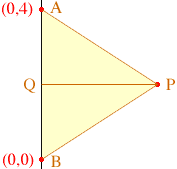An equilateral triangle is one in which all three sides are of equal length. If two vertices of an equilateral triangle are (0,4) and (0,0), find the third vertex. How many of these triangles are possible? Name: Shirley 12th grader Hi Shirley, Did you draw a diagram? You have two points (0,0) and (0,4) that are vertices of the triangle and you want a third vertex which will give an equilateral triangle. the point P in the diagram below.In the diagram I labeled the given points A and B, and Q is the midpoint of the linesegment AB. By the symmetry, triangles AQP and QBP are congruent and hence angle AQP is a right angle. Also since triangle ABP is equilateral, |AP|=4, and since Q is the midpoint of AB, |AQ|=2. Hence you can use Pythagoras' theorem to find the length of QP and hence the coordinates of P. Cheers, Penny Go to Math Central To return to the previous page use your browser's back button.